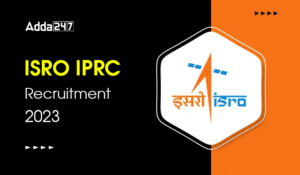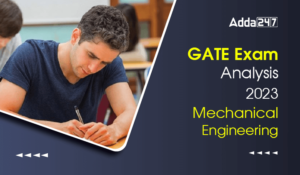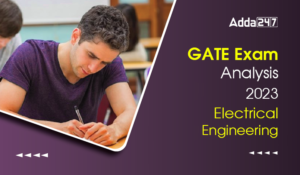Engineering Jobs   »   Mechincal Engineering quizs   »   NHPC JE Recruitment 2021, NHPC JE...

# NHPC JE’21 ME: Daily Practices Quiz. 29-Nov-2021

Quiz: Mechanical Engineering
Exam: NHPC JE
Topic: Miscellaneous

Each question carries 1 mark
Negative marking: 1/4 mark
Time: 6 Minutes

Q1. An open system
(a) is a specified region where transfers of energy and / or mass take place
(b) Is a region of constant mass and only energy is allowed to cross the boundaries
(c) Cannot transfer either energy or mass to or from the surroundings
(d) Has an enthalpy transfer across its boundaries and the mass within the system is not necessarily constant

Q2. Which pair of the following alternatives is correctly matched?
List – I List – II
(a) Heat – Point function
(b) Energy – Path function
(c) Entropy – Second law of thermodynamics
(d) Gibb’s function – Path function

Q3. The pressure difference (in Pa) in a droplet of a fluid of 0.002m diameter with surface tension of 0.01 N/m is
(a) 10
(b) 20
(c) 4 π
(d) 0.0004 π

Q4. A force of 400 N is required to open a process control valve. What is the area of diaphragm needed for a diaphragm actuator to open the valve with a control gauge pressure of 70 kPa?
(a) 0.0095 m^2
(b) 0.0086 m^2
(c) 0.0057 m^2
(d) 0.0048 m^2

Q5. Which of the following is correct to increase work ratio in a simple gas turbine power plant is?
1. Reheating
2. Regeneration
3. Intercooling
(a) 1, 2 and 3
(b) 2 and 3
(c) 1 and 3
(d) 1 and 2

Q6. What is the propulsion efficiency of a jet engine (where u is flight velocity and v is jet velocity relative to aircraft)
(a) 2u/(v-u)
(b) (v+u)/v
(c) 2u/(v+u)
(d) (v+u)/2v
Solutions

S1. Ans. (a)
In an open system both energy and mass transfer take place.
Examples of open system are Turbine, Pump, Compressor etc.

S2. Ans. (c)
Sol. Heat is a path function
Energy is a point function
Entropy is related with Second law of thermodynamics
Gibb’s function is a point function.

S3. Ans. (b)
Sol. ∆P=4σ/d=4×0.01/0.002=20 Pa

S4. Ans. (c)
Sol. Given that Force (F) = 400 N, Gauge Pressure (P) = 70 kPa
Area of diaphragm(A)
A=Force/Pressure=F/P=400/(70×1000)=0.0057 m^2

S5. Ans. (b)
Sol. Work ratio=W_net/W_turbune =(W_turbune-W_compressor)/W_turbune
In case of regeneration, there is no effect on work done. So, there is no effect on the work ratio.
In case of intercooling, the compressor work reduces, hence the work ratio increases.
In case of reheating, the turbine work increases, hence the work ratio increases.

S6. Ans. (c)
Sol. Propulsive efficiency of jet engine=2u/(v+u)

Sharing is caring!

•GATE 2023 Admit Card Out, Download Link ...
•GATE Exam Analysis 2023 Computer Science...
•GATE 2023 Memory Based Question Paper of...
•ISRO IPRC Recruitment 2023 Notification ...
•GATE Exam Analysis 2023 Mechanical Engin...
•GATE Exam Analysis 2023 Electrical Engin...
•NIT Warangal Recruitment 2023 Notificati...